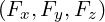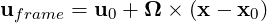### 3.5 Forcing

An optional section of the file allows forcing functions to be defined. These are enclosed in the `FORCING` tag. The forcing type is enclosed within the `FORCE` tag and expressed in the file as:

1<FORCE TYPE="[NAME]">
2    ...
3</FORCE>

The force type can be any one of the following.

#### 3.5.1 Absorption

This force type allows the user to apply an absorption layer (essentially a porous region) anywhere in the domain. The user may also specify a velocity profile to be imposed at the start of this layer, and in the event of a time-dependent simulation, this profile can be modulated with a time-dependent function. These velocity functions and the function defining the region in which to apply the absorption layer are expressed in the `CONDITIONS` section, however the name of these functions are defined here by the `COEFF` tag for the layer, the `REFFLOW` tag for the velocity profile, and the `REFFLOWTIME` for the time-dependent function.

1<FORCE TYPE="Absorption">
2    <COEFF> [FUNCTION NAME] <COEFF/>
3    <REFFLOW> [FUNCTION NAME] <REFFLOW/>
4    <REFFLOWTIME> [FUNCTION NAME] <REFFLOWTIME/>
5    <BOUNDARYREGIONS> 1,4 <BOUNDARYREGIONS/>
6</FORCE>

If a list of `BOUNDARYREGIONS` is specified, the distance to these regions is available as additional variable `r` in the definition of the `COEFF` function:

1<FUNCTION NAME="AbsorptionCoefficient">
2    <E VAR="p" EVARS="r" VALUE="-5000 * exp(-0.5 * (3*r / 0.4)^2)" />
3    <E VAR="u" EVARS="r" VALUE="-5000 * exp(-0.5 * (3*r / 0.4)^2)" />
4    <E VAR="v" EVARS="r" VALUE="-5000 * exp(-0.5 * (3*r / 0.4)^2)" />
5</FUNCTION>

#### 3.5.2 Body

This force type specifies the name of a body forcing function expressed in the `CONDITIONS` section.

1<FORCE TYPE="Body">
2    <BODYFORCE> [FUNCTION NAME] <BODYFORCE/>
3</FORCE>

#### 3.5.3 MovingReferenceFrame

This force type allows the solution of incompressilbe Navier-Stokes in moving frame of reference. The moving frame is attached the to body and can have translational, rotational or both motions. Although the Navier-Stokes equations are solved in a moving reference frame, our formulation is based on the absolute velocity and pressure (in inertial frame). However, note that these absolute velocities and any other vector quantities are expressed using the coordinate basis of the moving frame. Further, note that if you are using the FilterAeroForces, the force vectoris automatically converted and output in the inertial frame (ground reference frame).

To use this formulation the user need to specify the force type inside the `FORCING` tag as follwos:

1<FORCE TYPE="MovingReferenceFrame">
2    <LinearVelocity> [LinearVelocity FUNCTION NAME] <LinearVelocity/>
3    <AngularVelocity> [AngularVelocity FUNCTION NAME] <AngularVelocity/>
4    <PivotPoint> x0 y0 z0  <PivotPoint/>
5</FORCE>

Here we are required to provide at least one function for this force type which can be a function that defines the linear velocity of the reference frame or a function that defines the angular velocity of reference frame or both. In the case of rotating frame, i.e. when we are prescribing the angular velocity of reference frame, we can provide a coordinate of `PivotPoint`, around which the frame is rotating. If no pivot point provided, the orgin of coordinates in the moving reference frame will be used as the pivot point. Note that the frame velocities (both linear and angular velocities) must be defined in the inertial stationary frame of reference,i.e. ground reference frame (and expressed using the basis of inertial stationary frame), however, the Poivot point is in the moving reference frame.

Examples of linear and angular velocity funcitons together with their usage in the Forcing is shown below:

1<CONDITIONS>
2
3<FUNCTION NAME="LinVel">
4    <E VAR="u" VALUE="2*sin(PI*t)" />
5    <E VAR="v" VALUE="0.1" />
6    <E VAR="w" VALUE="0" />
7</FUNCTION>
8
9<FUNCTION NAME="AngVel">
10    <E VAR="Omega_x" VALUE="0" />
11    <E VAR="Omega_y" VALUE="0" />
12    <E VAR="Omega_z" VALUE="0.3*cos(2*PI*t)" />
13</FUNCTION>
14
15</CONDITIONS>
16
17<FORCING>
18
19    <FORCE TYPE="MovingReferenceFrame">
20        <LinearVelocity> LinVel <LinearVelocity/>
21        <AngularVelocity> AngVel <AngularVelocity/>
22        <PivotPoint> 0.2 0.0 0.0  <PivotPoint/>
23    </FORCE>
24
25</FORCING>

The moving frame functions defines the velocity of the body frame observed in the inertial reference frame. This means that these functions (such as the `LinVel` and `AngVel` in the above example) are defined and expressed in the stationary inertial frame (ground frame).

Here, u0 = (u, v, w) is the translational velocity, Ω = (Omega_x, Omega_y, Omega_z) is the angular velocity. x0 = (0.2,0.0,0.0) is the rotation pivot and it is fixed in the body frame. Translational motion is allowed for all dimensions while rotational motion is currently restricted to z (omega_z) for 2D, 3DH1D and full 3D simulaitons.

Finally, note that when using `MovingReferenceFrame` force type, for any open part of the computational domain that the user specifies the velocity, such as inlet and free stream boundary conditions, the `USERDEFINEDTYPE="MovingFrameDomainVel"` tag should be used for all of velocity components. For example if boundary `ID=2` is the inlet with `Uinfx` and `Uinfy` the values of inlet velocities defined as parameters, the boundary condition for this boundary becomes:

1<REGION REF="2">
2   <D VAR="u" USERDEFINEDTYPE="MovingFrameDomainVel" VALUE="Uinfx" />
3   <D VAR="v" USERDEFINEDTYPE="MovingFrameDomainVel" VALUE="Uinfy" />
4   <N VAR="p" USERDEFINEDTYPE="H" VALUE="0" />
5</REGION>

for the wall boundary conditions on the surface of the body, we need to use `MovingFrameWall` tag as shown below:

1<REGION REF="0">
2    <D VAR="u" USERDEFINEDTYPE="MovingFrameWall" VALUE="Uinfx" />
3    <D VAR="v" USERDEFINEDTYPE="MovingFrameWall" VALUE="Uinfy" />
4    <N VAR="p" USERDEFINEDTYPE="H" VALUE="0" />
5</REGION>

The outlet and pressure boundary conditions are the same as before.

#### 3.5.4 Programmatic

This force type allows a forcing function to be applied directly within the code, thus it has no associated function.

1<FORCE TYPE="Programmatic">
2</FORCE>

#### 3.5.5 Noise

This force type allows the user to specify the magnitude of a white noise force. Optional arguments can also be used to define the frequency in time steps to recompute the noise (default is never) and the number of time steps to apply the noise (default is the entire simulation).

1<FORCE TYPE="Noise">
2    <WHITENOISE> [VALUE] <WHITENOISE/>
3    <!-- Optional arguments -->
4    <UPDATEFREQ> [VALUE] <UPDATEFREQ/>
5    <NSTEPS> [VALUE] <NSTEPS/>
6</FORCE>